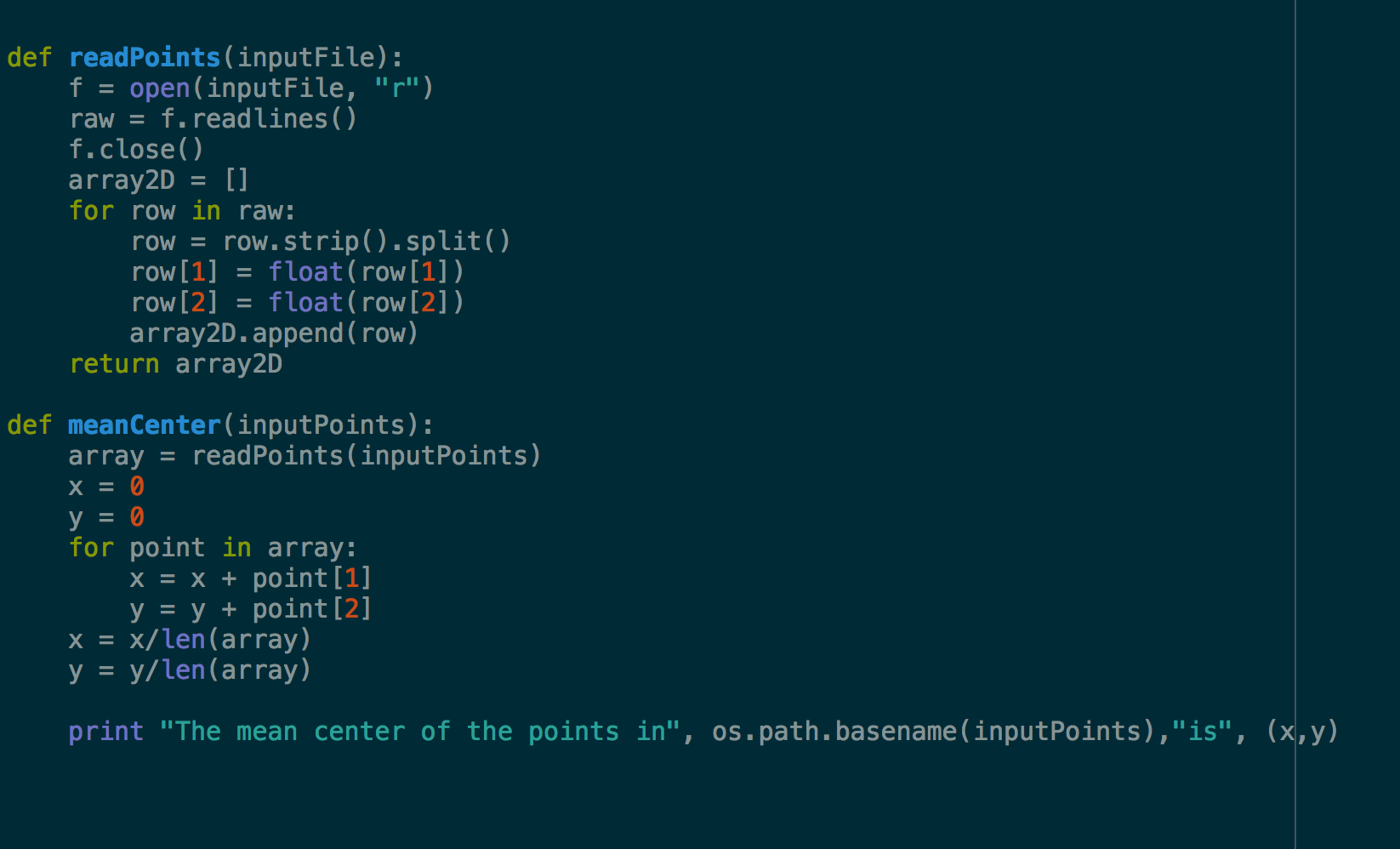# How do you use python to calculates mean center of multiple shapefiles located in one folder?

3408
4
06-24-2016 03:56 PMNew Contributor

I am trying to write a script that will calculate multiple mean centers of a group of shapefiles.  I have multiple shapefiles that each need a mean center calculated.

Tags (3)
4 RepliesbyMVP Esteemed Contributor

We shall rule out the center of the file extent obviously.

And you have looked at the tools in here ... An overview of the Spatial Statistics toolbox—Help | ArcGIS for Desktop

What type of features are they? Points, polylines or polygons?

The reason why I ask is because if it is the latter two, you are going to get a different value if you use all the points in a poly* feature than if you take mean center of the feature centers.  This will obviously compounded by the introduction of multiple input files.

So having said that, how adept are you at scripting since both options can be done using numpy which will save you a load of searchcursor stuff. having said that you can calculate the centroids of your poly* features then get a field summary for each file.  There are other options, but what you actually need will determine what method you should use.New Contributor

This might do the trick:New Contributor

or just:

import arcpy

meancenter = MeanCenter_stats (Input_Feature_Class, Output_Feature_Class, {Weight_Field}, {Case_Field}, {Dimension_Field})byEsri Esteemed Contributor

If you don't want to program, just merge the shapefiles into a single feature class and use the Mean Center—Help | ArcGIS Desktop (in case you want to obtain 1 mean center of all the points you have in multiple shapefiles)

If you prefer using code you could use something like this and assuming that all shapefiles have the same coordinate system:

``def main():    import arcpy    import os    ws = r'C:\Folder\Where\Shapefiles\Are\Stored'    fc_out = r'C:\Folder\Where\Shapefiles\Are\Stored\MeanCenter.shp'    # create list of featureclasses    arcpy.env.workspace = ws    lst_fc = arcpy.ListFeatureClasses()    # loop through each featureclass and create list if (x,y)    lst_all_xy = []    for fc_name in lst_fc:        fc = os.path.join(ws, fc_name)        lst_xy = [r for r in arcpy.da.SearchCursor(fc, ('SHAPE@XY'))]        lst_all_xy.extend(lst_xy)    # create mean center    if len(lst_all_xy) > 0:        lst_x = [xy for xy in lst_all_xy]        lst_y = [xy for xy in lst_all_xy]        pnt = arcpy.Point(sum(lst_x)/float(len(lst_x)), sum(lst_y)/float(len(lst_y)))        sr = arcpy.Describe(fc).spatialReference        pntg = arcpy.PointGeometry(pnt, sr)        arcpy.CopyFeatures_management([pntg], fc_out)if __name__ == '__main__':    main()‍‍‍‍‍‍‍‍‍‍‍‍‍‍‍‍‍‍‍‍‍‍‍‍‍‍‍‍‍‍‍‍‍‍‍‍‍‍‍‍‍‍‍‍‍‍‍‍‍‍‍‍‍‍‍‍‍‍``

Change the paths on line 5 and 6